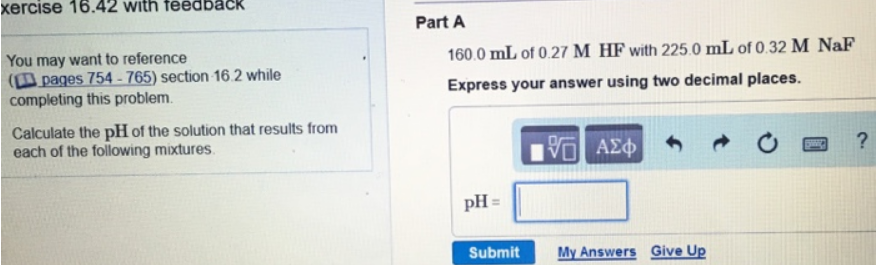# Problem: Calculate the pH of the solution that results from each of the following mixtures. Part A160.0 mL of 0.27 M HF with 225.0 mL of 0.32 M NaF.Express your answer using two decimal places.

###### FREE Expert Solution
98% (165 ratings)###### Problem Details

Calculate the pH of the solution that results from each of the following mixtures.

Part A

160.0 mL of 0.27 M HF with 225.0 mL of 0.32 M NaF.